# Micro Aerial Vehicle Trail Navigation Nets Trained onIDSIA Swiss Alps and PASCAL VOC Data

Navigate a drone in a forest environment

Released in 2017, these two models constitute a system for autonomous path navigation in unstructured, outdoor environments such as forests. Specifically, this model is trained for steering in a forest environment. The system consists of two main submodules: a navigation net (TrailNet DNN) and an obstacle detection net. The navigation net is a two-headed classifier used to estimate rotation directions and lateral translations given an input image, with a total of three categories each. These output probabilities are later combined to predict a final rotation angle. It is based on ResNet-18, with batch normalization layers removed and ReLUs replaced with shifted ReLUs.

The obstacle detection net is an object detection model based on YOLO V1 with a few modifications, such as removal of batch normalizations and replacement of leaky ReLUs by ReLUs. In case a detected object occupies a large proportion of the space within the image frame, the vehicle is forced to stop.

Number of models: 2

## Training Set Information

• The TrailNet DNN was first trained on the IDSIA dataset to learn the rotation directions and then on a private unreleased dataset collected in the Pacific Northwest to predict translation offsets. The IDSIA dataset is a dataset for the visual perception of forest trails consisting of 17,119 training images and 7,355 test images, with three classes of objects. The private dataset used to learn the translation offsets consists of footages of hikes recorded by three cameras capturing a diversity of cross-seasonal landscape scenes. The object detection net was retrained on the PASCAL VOC datasets, presumably on a combination of PASCAL VOC 2007 and PASCAL VOC 2012.

## Examples

### Resource retrieval

Get the pre-trained net:

 In:=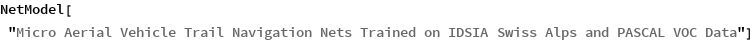Out=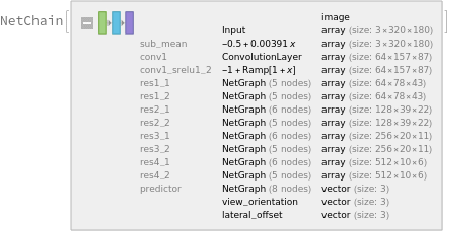### NetModel parameters

This model consists of a family of individual nets, each identified by a specific parameter combination. Inspect the available parameters:

 In:=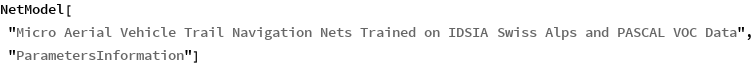Out=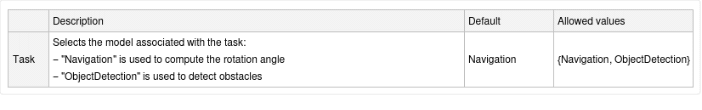Pick a non-default net by specifying the parameter:

 In:=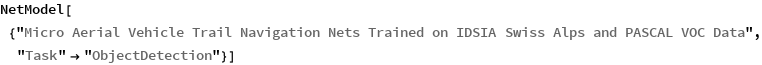Out=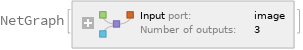Pick a non-default uninitialized net:

 In:=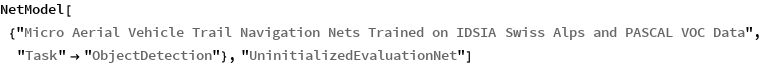Out=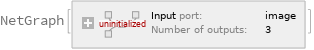### Evaluation functions

#### Evaluation function for the navigation model

Define an evaluation function to calculate the turning angle in radians:

 In:=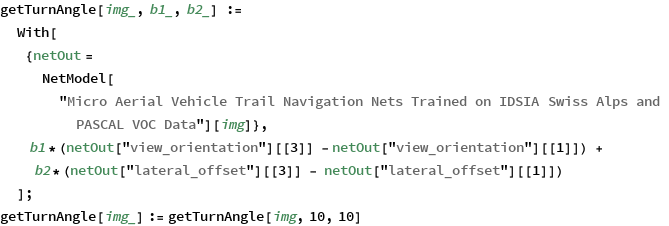#### Evaluation function for object detection model

Write an evaluation function to scale the result to the input image size and suppress the least probable detections:

 In:=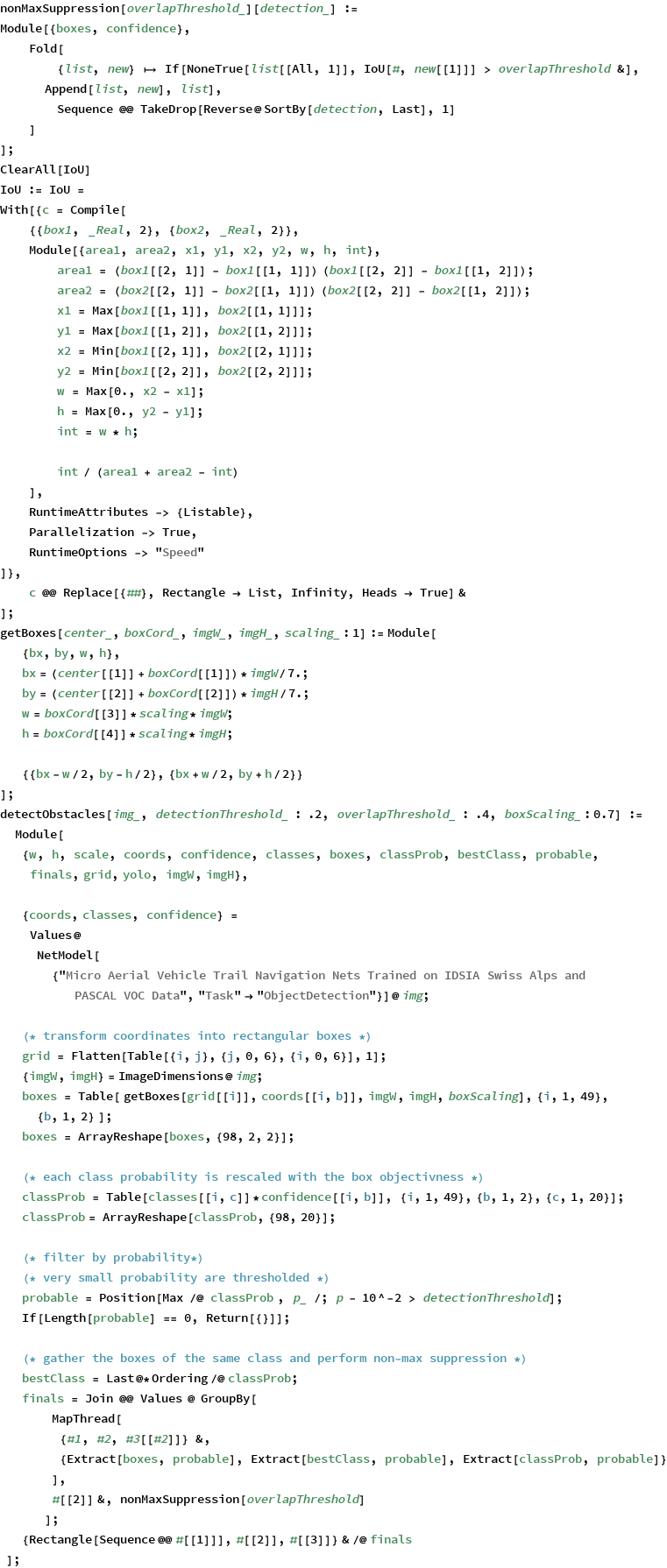### Label list for the object detection model

Define the label list for this model. Integers in the model's output correspond to elements in the label list:

 In:=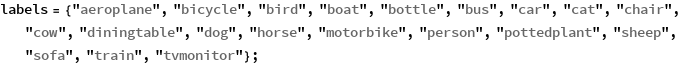Calculate the turn angle in radians given a test image:

 In:=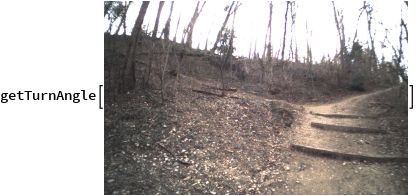Out=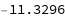### Basic usage: object detection model

Obtain the detected bounding boxes with their corresponding classes and confidences for a given image:

 In:=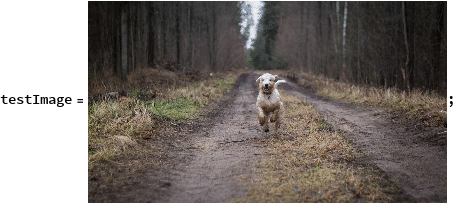In:=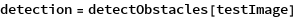Out=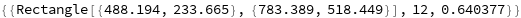Inspect which classes are detected:

 In:=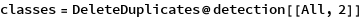Out=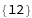In:=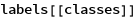Out=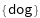Visualize the detection:

 In:=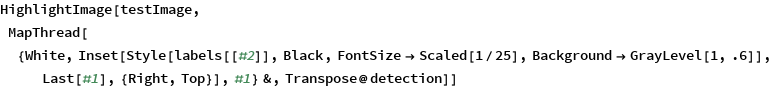Out=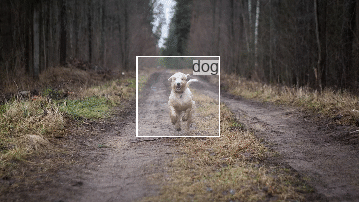### Detection results

Define an image:

 In:=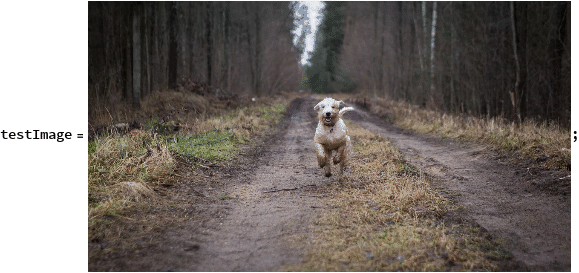The network computes 98 bounding boxes and the probability that the objects in each box are of any given class:

 In:=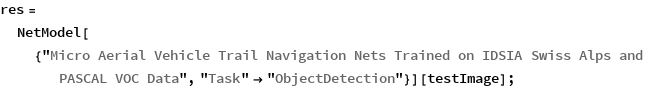In:=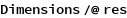Out=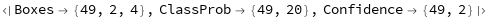Visualize all the boxes predicted by the net scaled by their "Confidence" measures:

 In:=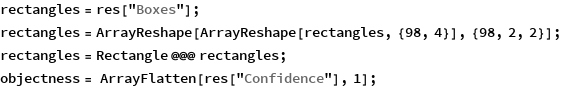In:=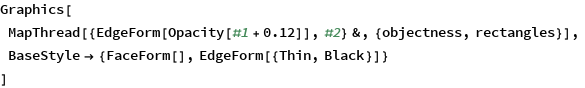Out=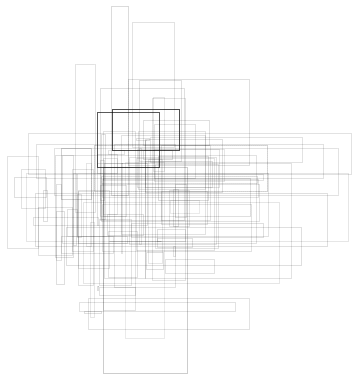### Net information

Inspect the number of parameters of all arrays in the net:

 In:=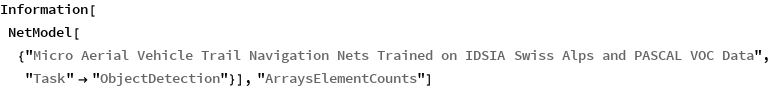Out=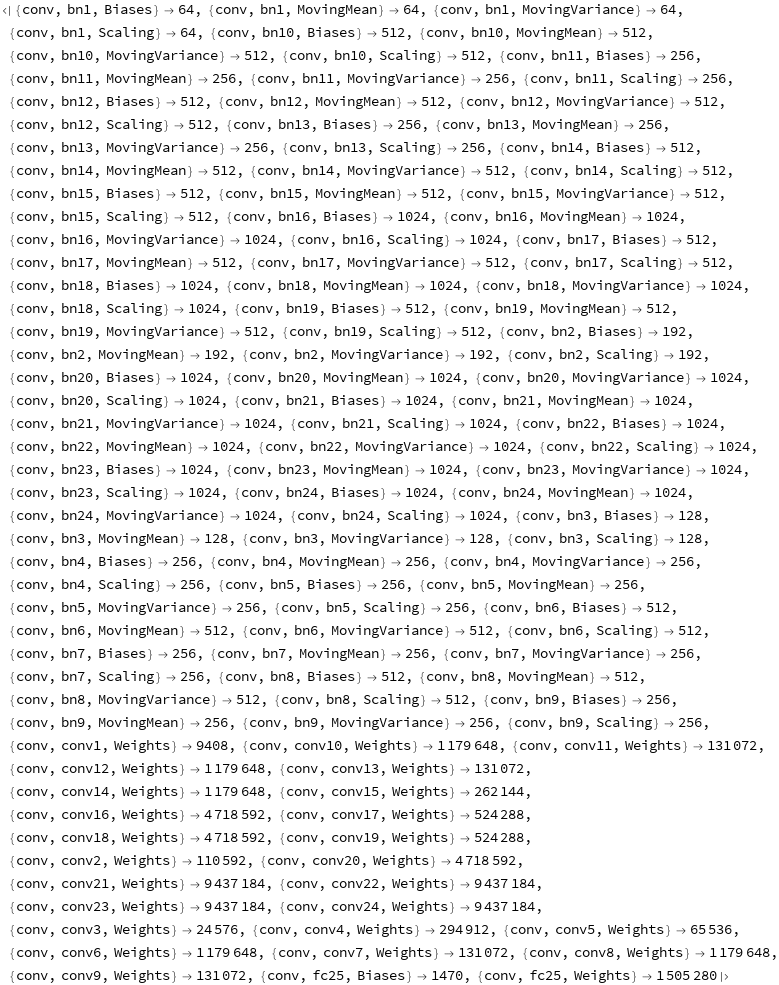In:=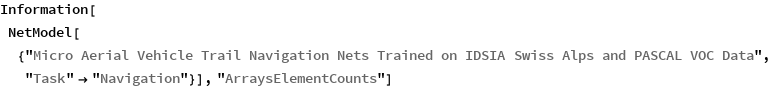Out=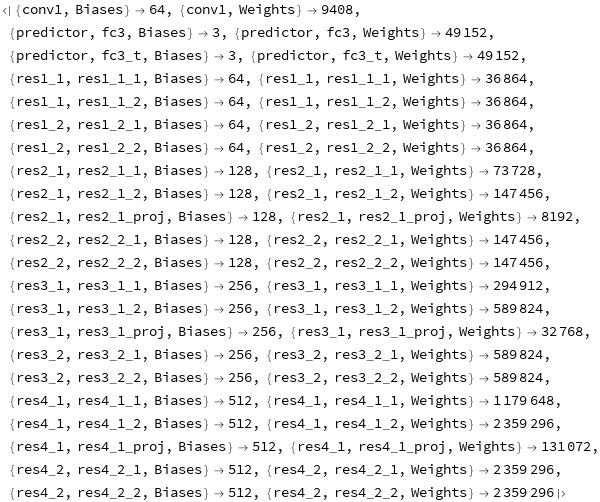Obtain the total number of parameters:

 In:=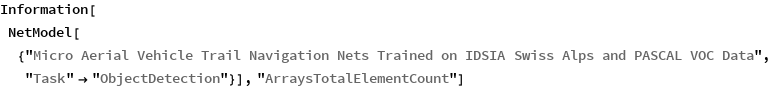Out=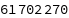In:=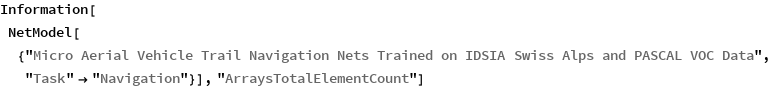Out=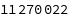Obtain the layer type counts:

 In:=Out=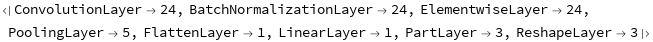In:=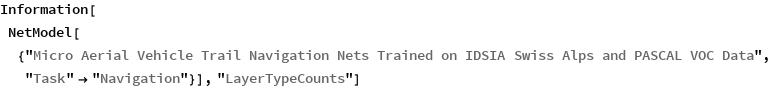Out=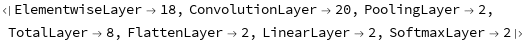Display the summary graphic:

 In:=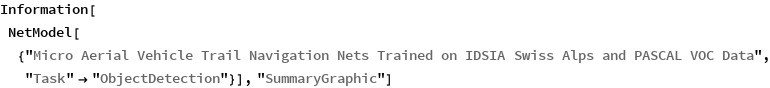Out=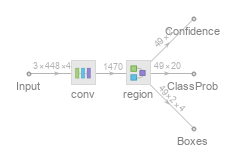In:=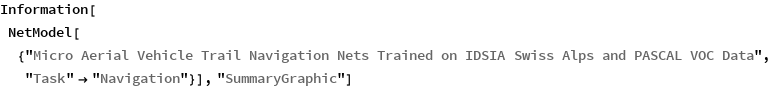Out=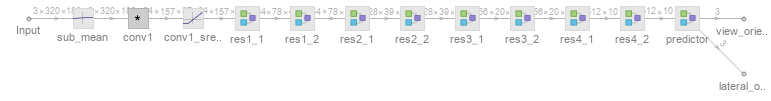### Export to MXNet

Export the nets into a format that can be opened in MXNet:

 In:=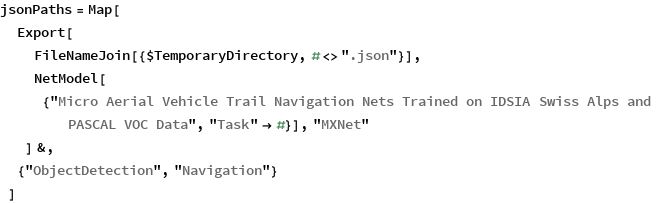Out=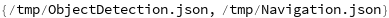Export also creates a net.params file containing parameters:

 In:=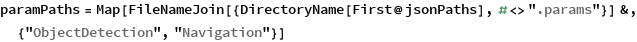Out=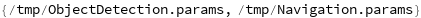Get the size of the parameter files:

 In:=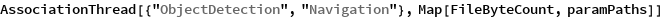Out=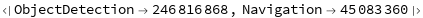## Reference

• N. Smolyanskiy, A. Kamenev, J. Smith, S. Birchfield, "Toward Low-Flying Autonomous MAV Trail Navigation Using Deep Neural Networks for Environmental Awareness," arXiv:1705.02550 (2017)
• (available from https://github.com/NVIDIA-AI-IOT/redtail)